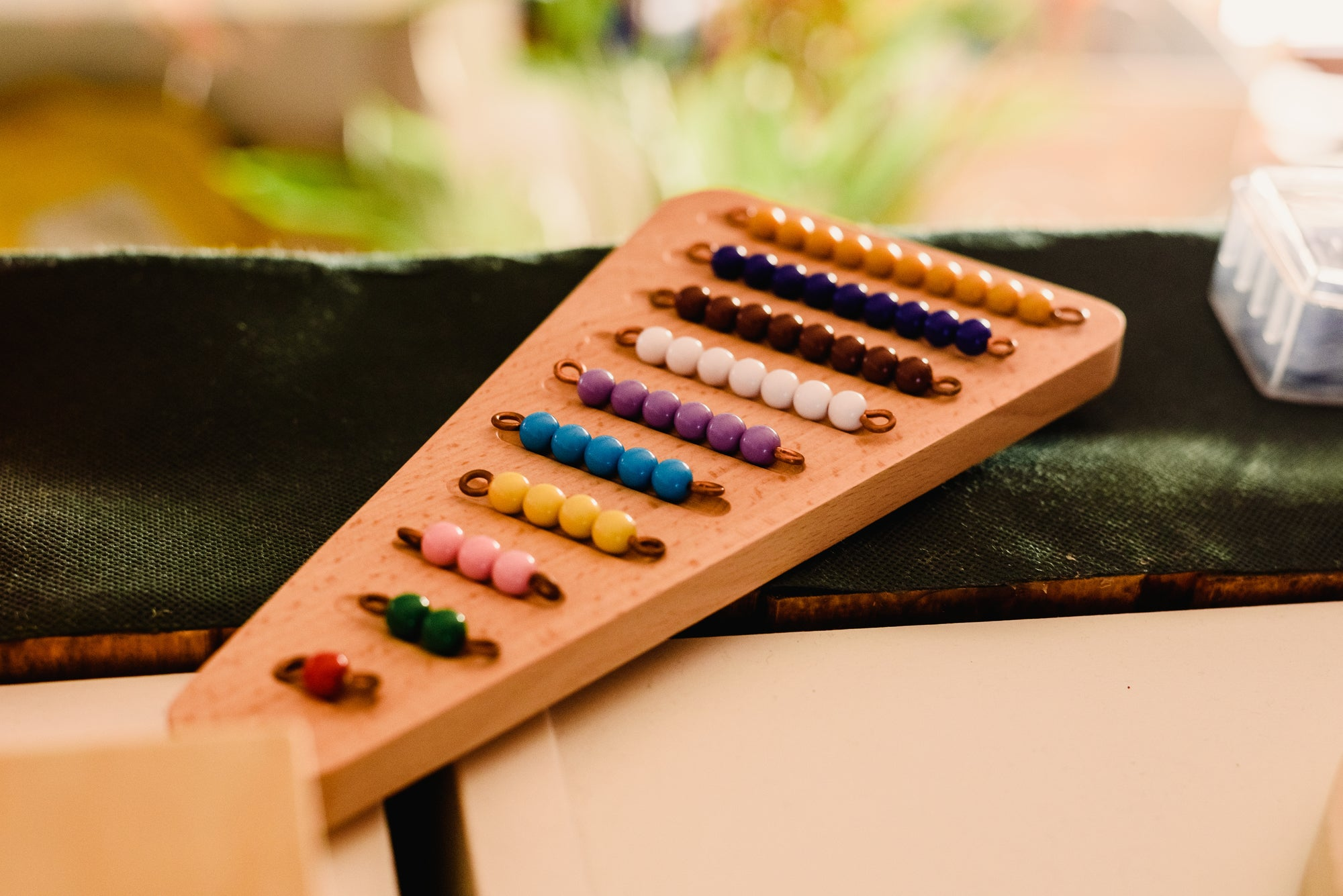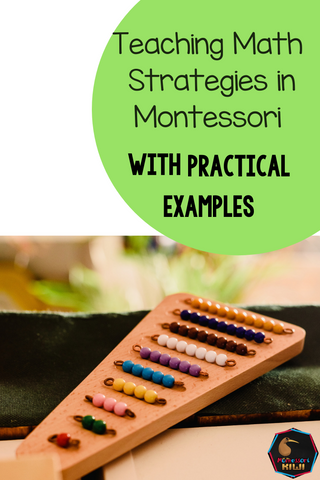# Teaching maths strategies in a Montessori 6-12 classI know many Montessorians who love the Montessori maths materials. In particular how they make abstract concepts real. Take for example the fraction circles that allow the child to touch and experiment with different amounts to find out equivalents and to see how one size differs to the other.

One thing that I feel we need to work on more with our Maths teaching is how we teach strategy. Here in New Zealand we have been taught a lot about different strategies children can use and as teachers we have been encouraged to help children learn different strategies.

Just like maths knowledge strategy has to be taught and opportunities need to be given for the child to solve using a strategy.

Let’s take for example a child who knows their times tables for 6 x 4 or six groups of four. Imagine this child is laying out 6 groups of 4 yellow beads on the checkerboard and instead of using their times tables counts the beads in groups 4, 8, 12,16, 20, 24. This child may know their times tables in one context , say using the multiplication charts but doesn’t transfer this to a different situation. As teachers we can encourage the child to do so.

A child may be given an equation to solve  with the stamp game that says 26  + 14 . After the child has solved it with the stamp game successfully we might introduce another way the child could solve the equation for example  by using place value and  going (20 + 10) + (6 + 4)  or by using a tidy number and compensation going 30 + 10

Here is a quick (not exhaustive look) at some strategies a child may go through.  These are based on the NZ Numeracy project. Just like the Montessori equipment a child doesn’t need to know every strategy however we need to have a knowledge of these things so that we can help students see connections.

Students learn the operations (addition, subtraction, multiplication and division) alongside each other and at different rates so a child may have a more advanced addition strategy than a multiplication strategy.

When a student 'knows' something as opposed to having to work something out that is no longer a strategy but knowledge.

Just like the Montessori philosophy teaches us at a younger age or lower level students may be more reliant on equipment to teach them a strategy where as at an older age or more advanced strategy level they may be able to work out something in their head.

The lower down the list the more complicated the strategy.

ADDITION (students begin with this set of strategies, note other operations strategies will develop a little bit later but alongside addition)

• Knows how to count from 1 to work out how many of an object there are e.g. counts from 1 to work out how many of a coloured bead there are. Note that a student will soon recognise that four yellows are four and this is then number knowledge not a strategy as a student just 'knows' this.
• Puts objects into groups to work out how many of something there are
• Counts everything (may use fingers or material or do in their head) to work out the answer in an equation such as 7 + 4 for example goes 1,2,3,4,5,6,7 ….. 8,9,10,11,12
• Counts on in their head e.g. doesn’t need materials to work out 37 + 9. The student goes 37,38……. 46 (may still revert to fingers though!)
• Uses doubles to work out a nearby basic fact e.g. knows to solve 7 + 8 they could go 7 + 7 + 1 or 8 + 8 – 1
• Makes a ten (finds a nearby tidy number, a tidy number is one that ends in 0) e.g. knows 38 + 6 could be solved as 38 + 2 = 40 + 4 = 44
• Place value partioning with two digits 32 + 24   = (30 + 20) + (2+ 4). You will note how this is different to how we teach adding with equipment where we have students add from the units then do the tens, however students should not be discouraged from using place value partioning.
• Place value partioning with three digits
• Using tidy numbers and compensating to help solve an equation e.g. 89 + 21 is like 90 + 20
• Splits fractions and uses equivalent fractions ¾ + 6/8 is like saying 6/8 + 6/8. Adds to onto 6/8 to make a whole and then adds on the rest
• Uses place value, reversing and tidy numbers with decimals eg. applies the strategies from whole numbers to decimal numbers
• Able to convert different amounts e.g. percentages, decimals and decimals and add them together to work out an equation.

SUBTRACTION

• Splits a group of objects to find out how many are left over
• Counts everything (may use fingers or material or do in their head) to work out the answer in an equation such as 12- 3 as 12,11,10,9
• Counts back in their head e.g. doesn’t need materials to work out 56-8. The student goes 56,55,54 all the way to 48.
• Uses place value to solve an equation 734 – 106 as 734 – 100 – 6
• Uses tidy numbers 834- 479 as 834 – 500 + 21
• Reverses an equation to find out the answer e.g. 723 – 342 as 342 + ___ = 723
• Uses place value, reversing and tidy numbers with decimals eg. applies the strategies from whole numbers to decimal numbers

MULTIPLICATION

• Uses repeated addition (often evident on the multiplication bead board) counts 4 + 4 + 4 + 4 to work out 4 x 4
• Knows to reverse multiplications around to work out an equation from a known fact e.g. 6 x 5 is the same as 5 x 6.
• Uses place value to split an equation e.g. 72 x 5 can be solved by going (70 x 5) + (2 x 5 )
• Uses place value, reversing and compensating from tidy numbers with decimals (note that this is harder than adding and subtracting so may occur later)

DIVISION

• Knows how a division fact relates to a multiplication fact
• May split a number by cutting in half e.g. 48 divided by 4 as 48 divided by 2 then dividing by 2 again
• Uses place value 168 divided by 7 as 140 / 7 = 20, 28 / 7 = 4, 20 + 8 = 28
• Compensating from a tidy number by using a relevant basic fact e.g. 208 / 7 as 210 / 7 then taking off the ‘extra’
• Uses place value, reversing and compensating from tidy numbers with decimals (note that this is harder than adding and subtracting so may occur later)

To find out what maths knowledge your student knows and what strategies they are using I have designed this maths assessment. It is not designed to grade students but to assist you with your next presentations and lessons. It clearly states what the question is assessing and gives ideas of next lessons you might give.You can find more of my maths resources here

These ones have been designed to encourage students maths strategies

Interested in More Montessori Curriculum resource blog posts? Find them here

You can join my mailing list to get more Montessori resources, notifications of new products and news here

## 1 comment

Hi, thank you very much for writng this article. Now, I am more able to marry Montessori Maths knowledge and strategies for the benefit of the children in the classroom.
Regards,
Rani

Rani